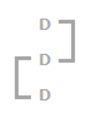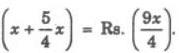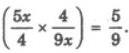# Arithmetical Reasoning MCQ

## 25 Questions MCQ Test Logical Reasoning for CLAT | Arithmetical Reasoning MCQ

Description
This mock test of Arithmetical Reasoning MCQ for CLAT helps you for every CLAT entrance exam. This contains 25 Multiple Choice Questions for CLAT Arithmetical Reasoning MCQ (mcq) to study with solutions a complete question bank. The solved questions answers in this Arithmetical Reasoning MCQ quiz give you a good mix of easy questions and tough questions. CLAT students definitely take this Arithmetical Reasoning MCQ exercise for a better result in the exam. You can find other Arithmetical Reasoning MCQ extra questions, long questions & short questions for CLAT on EduRev as well by searching above.
QUESTION: 1

### A shepherd had 17 sheep. All but nine died. How many was he left with?

Solution:

'All but nine died' means 'All except nine died' i.e. 9 sheep remained alive.

QUESTION: 2

### A bird shooter was asked how many birds he had in the bag. He replied that there were all sparrows but six, all pigeons but six, and all ducks but six. How many birds he had in the bag in all?

Solution:

There were all sparrows but six' means that six birds were not sparrows but only pigeons and ducks.
Similarly, number of sparrows + number of ducks = 6 and number of sparrows + number of pigeons = 6.
This is possible when there are 3 sparrows, 3 pigeons and 3 ducks i.e. 9 birds in all.

QUESTION: 3

### What is the smallest number of ducks that could swim in this formation—two ducks in front of a duck, two ducks behind a duck and a duck between two ducks?

Solution:

Clearly, the smallest such number is 3.Three ducks can be arranged as shown above to satisfy all the three given conditions.

QUESTION: 4

The 30 members of a club decided to play a badminton singles tournament. Every time a member loses a game he is out of the tournament. There are no ties. What is the minimum number of matches that must be played to determine the winner?

Solution:

Clearly, every member except one (i.e. the winner) must lose one game to decide the winner. Thus, minimum number of matches to be played = 30 - 1 = 29.

QUESTION: 5

A man wears socks of two colours—black and brown. He has altogether 20 black socks and 20 brown socks in a drawer. Supposing he has to take out the socks in the dark, how many must he take out to be sure that he has a matching pair?

Solution:

Since there are socks of only two colours, so two out of any three socks must always be of the same colour.

QUESTION: 6

What is the product of all the numbers in the dial of a telephone?

Solution:

Since one of the numbers on the dial of a telephone is zero, so the product of all the numbers on it is 0.

QUESTION: 7

A group of 1200 persons consisting of captains and soldiers is travelling in a train. For every 15 soldiers there is one captain. The number of captains in the group is

Solution:

Generally we may commit mistake of dividing 1200/15.
But out of 16 persons there is one captain.
so, it will be 1200/16 = 75

QUESTION: 8

Aruna cut a cake into two halves and cuts one half into smaller pieces of equal size. Each of the small pieces is twenty grams in weight. If she has seven pieces of the cake in all with her, how heavy was the original cake?

Solution:

Seven pieces of cakes means one half cake and six pieces of another half cake
Total weight of 6 small pieces =6 x 20 g=120g
∴ Weight of the half cake=120 g
Total weight of the cake=240 g.

QUESTION: 9

First bunch of bananas has 1/4 again as many bananas as a second bunch. If the second bunch has 3 bananas less than the first bunch, then the number of bananas in the-first bunch is

Solution:

Assuming the number of bananas in the second bunch to be x
No. of bananas in 1st is x + x/4 = 5x/4
So, 5x/4 - x = 3x/4 = 3
x = 12
No. of bananas in 1st = 5x/4 = 5 x 12/4 = 5 x 3 = 15

QUESTION: 10

A tailor had a number of shirt pieces to cut from a roll of fabric. He cut each roll of equal length into 10 pieces. He cut at the rate of 45 cuts a minute. How many rolls would be cut in 24 minutes ?

Solution:

It is quite clear that the number of cuts made to cut a roll into 10 pieces = 9.
So, required number of rolls = (45 x 24)/9 = 120 rolls
Hence, option (D) is the correct answer.

QUESTION: 11

In a class of 60 students, the number of boys and girls participating in the annual sports is in the ratio 3 : 2 respectively. The number of girls not participating in the sports is 5 more than the number of boys not participating in the sports. If the number of boys participating in the sports is 15, then how many girls are there in the class?

Solution:

Let the number of boys and girls participating in sports be 3x and 2x respectively.
Then, 3x = 15 or x = 5.
So, number of girls participating in sports = 2x = 10.
Number of students not participating in sports = 60 - (15 + 10) = 35.
Let number of boys not participating in sports be y.
Then, number of girls not participating in sports = (35 -y).
Therefore (35 - y) = y + 5 <=> 2y<=>  30<=> y = 15.
So, number of girls not participating in sports = (35 - 15) = 20.
Hence, total number of girls in the class = (10 + 20) = 30.

QUESTION: 12

In a class, there are 18 boys who are over 160 cm tall. If these constitute three-fourths of the boys and the total number of boys is two-thirds of the total number of students in the class, what is the number of girls in the class?

Solution:

Let the number of boys be x.
Then, 3/4 x = 18
=> x = 24
If total number of students is y, then
2/3 y = 24
=> y = 36
Number of girls in class = y - x
= 36 - 24 = 12

QUESTION: 13

A student got twice as many sums wrong as he got right. If he attempted 48 sums in all, how many did he solve correctly?

Solution:

Let us suppose right sums are x then according to question wrong sums will be 2x and we know if we sum up right ones with the wrong then we will get no of questions attempted ' 2x+x = 48 then we will get value of x= 16

QUESTION: 14

The number of boys in a class is three times the number of girls. Which one of the following numbers cannot represent the total number of children in the class?

Solution:

Let number of girls = x and number of boys = 3x.
Then, 3x + x = 4x = total number of students.
Thus, to find exact value of x, the total number of students must be divisible by 4. 42 is not divisible by 4.

QUESTION: 15

A placed three sheets with two carbons to get two extra copies of the original. Then he decided to get more carbon copies and folded the paper in such a way that the upper half of the sheets were on top of the lower half. Then he typed. How many carbon copies did he get?

Solution:

Since the number of carbons is 2, only two copies can be obtained.

QUESTION: 16

A motorist knows four different routes from Bristol to Birmingham. From Birmingham to Sheffield he knows three different routes and from Sheffield to Carlisle he knows two different routes. How many routes does he know from Bristol to Carlisle?

Solution:

Total number of routes from Bristol to Carlisle =(4×3×2)=24.

QUESTION: 17

A pineapple costs Rs. 7 each. A watermelon costs Rs. 5 each. X spends Rs. 38 on these fruits. The number of pineapples purchased is

Solution:

Let the number of pineapples and watermelons be x and y respectively.
Then, 7x+5y=38 or 5y=(38−7x)
or y= 5
38−7x
​Clearly, y is a whole number, only when (38−7x) is divisible by 5.
This happens when x=4.

QUESTION: 18

If you write down all the numbers from 1 to 100, then how many times do you write 3?

Solution:

Clearly, from 1 to 100, there are ten numbers with 3 as the unit's digit- 3, 13, 23, 33, 43, 53, 63, 73, 83, 93; and ten numbers with 3 as the ten's digit - 30, 31, 32, 33, 34, 35, 36, 37, 38, 39.

So, required number = 10 + 10 = 20.

QUESTION: 19

The total number of digits used in numbering the pages of a book having 366 pages is

Solution:

Total number of digits
= (No. of digits in 1- digit page nos. + No. of digits in 2-digit page nos. + No. of digits in 3- digit page nos.)
= (1 x 9 + 2 x 90 + 3 x 267) = (9 + 180 + 801) = 990.

QUESTION: 20

A printer numbers the pages of a book starting with 1 and uses 3189 digits in all. How many pages does the book have?

Solution:

No. of digits in 1-digit page nos. = 1x9 = 9.
No. of digits in 2-digit page nos. = 2 x 90 = 180.
No. of digits in 3-digit page nos. = 3 x 900 = 2700.
No. of digits in 4-digit page nos. = 3189 - (9 + 180 + 2700) = 3189 - 2889 = 300.
Therefore No. of pages with 4-digit page nos. = (300/4) = 75.
Hence, total number of pages = (999 + 75) = 1074.

QUESTION: 21

In a garden, there are 10 rows and 12 columns of mango trees. The distance between the two trees is 2 metres and a distance of one metre is left from all sides of the boundary of the garden. The length of the garden is

Solution:

Each row contains 12 plants.

There are 11 gapes between the two corner trees (11 x 2) metres and 1 metre on each side is left.

Therefore Length = (22 + 2) m = 24 m.

QUESTION: 22

A farmer built a fence around his square plot. He used 27 fence poles on each side of the square. How many poles did he need altogether?

Solution:

Since each pole at the corner of the plot is common to its two sides, so we have :

Total number of poles needed = 27 x 4 - 4 = 108 - 4 = 104.

QUESTION: 23

An enterprising businessman earns an income of Re. 1 on the first day of his business. On every subsequent day, he earns an income which is just double of that made on the previous day. One the 10th day of business, his income is

Solution:

Income on the first day = Re. 1.
Income on the 2nd day =
Rs. (1×2)= Rs. 21
Income on the 3rd day =
Rs. (21×2)= Rs.22
and so on. Thus, Income on the rth day = Rs. 2n−1
.Therefore Income on the 10th day = Rs. 29
.

QUESTION: 24

In a family, the father took 1/4  of the cake and he had 3 times as much as each of the other members had. The total number of family members is

Solution:

Let the family members b x.
Father ate 1/4 of the cake;
Rest members got 3/4x,
the father had 3 times more than other members share i.e 3/4,
Now,
3(3/4x) = 1/4,
3/4x = 1/12,
36 = 4x,
X = 9,
9 + 1(father) = 10 total family member.

QUESTION: 25

A waiter’s salary consists of his salary and tips. During one week his tips were 5/4  of his salary. What fraction of his income came from tips?

Solution:

Let Salary = Rs. x. Then tips = Rs. ((5/4)x).
Total income = Rs.∴ Required fraction =